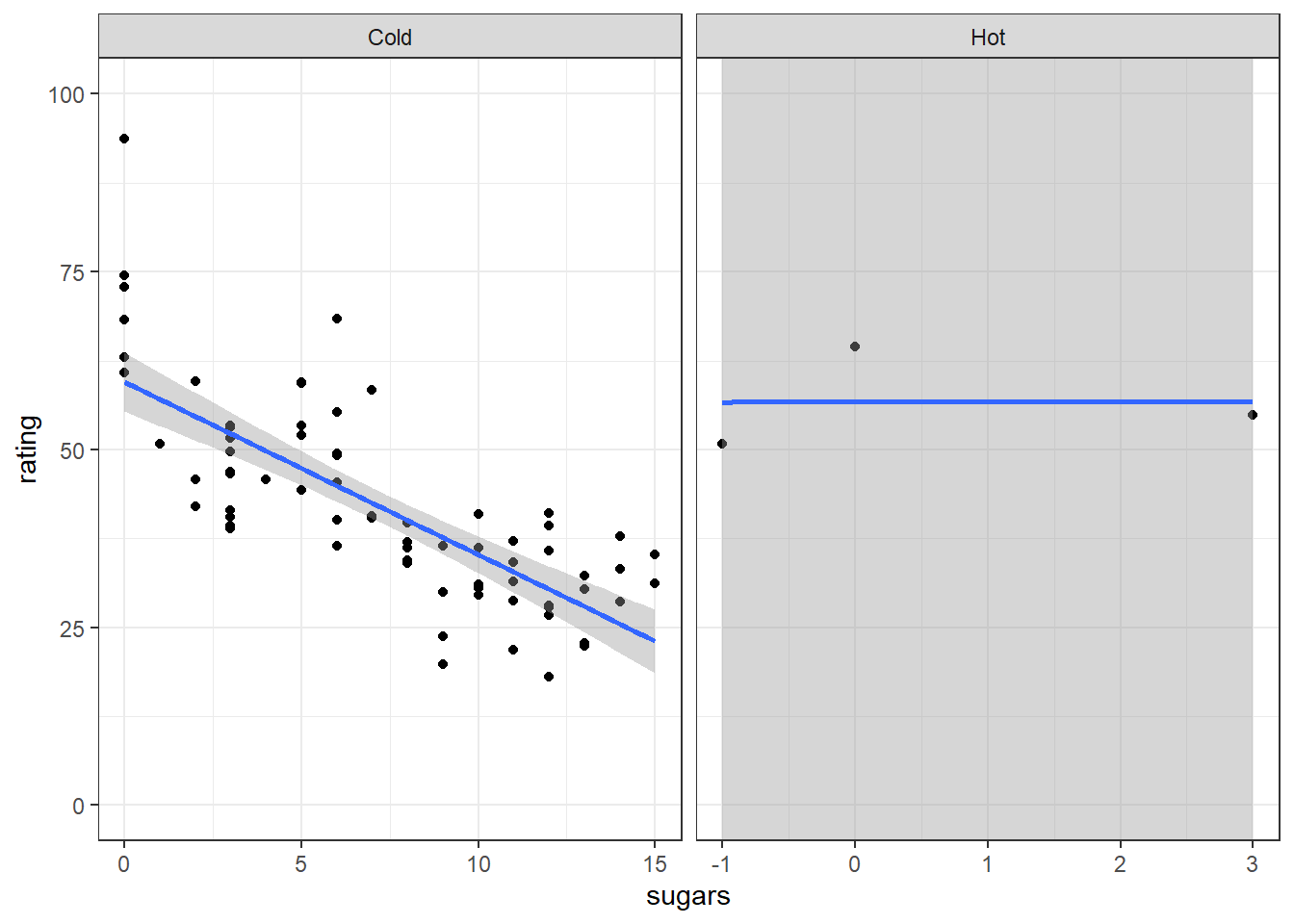### Exercise 1

#### 1a

ggplot(cereal, aes(x = rating)) +
geom_density()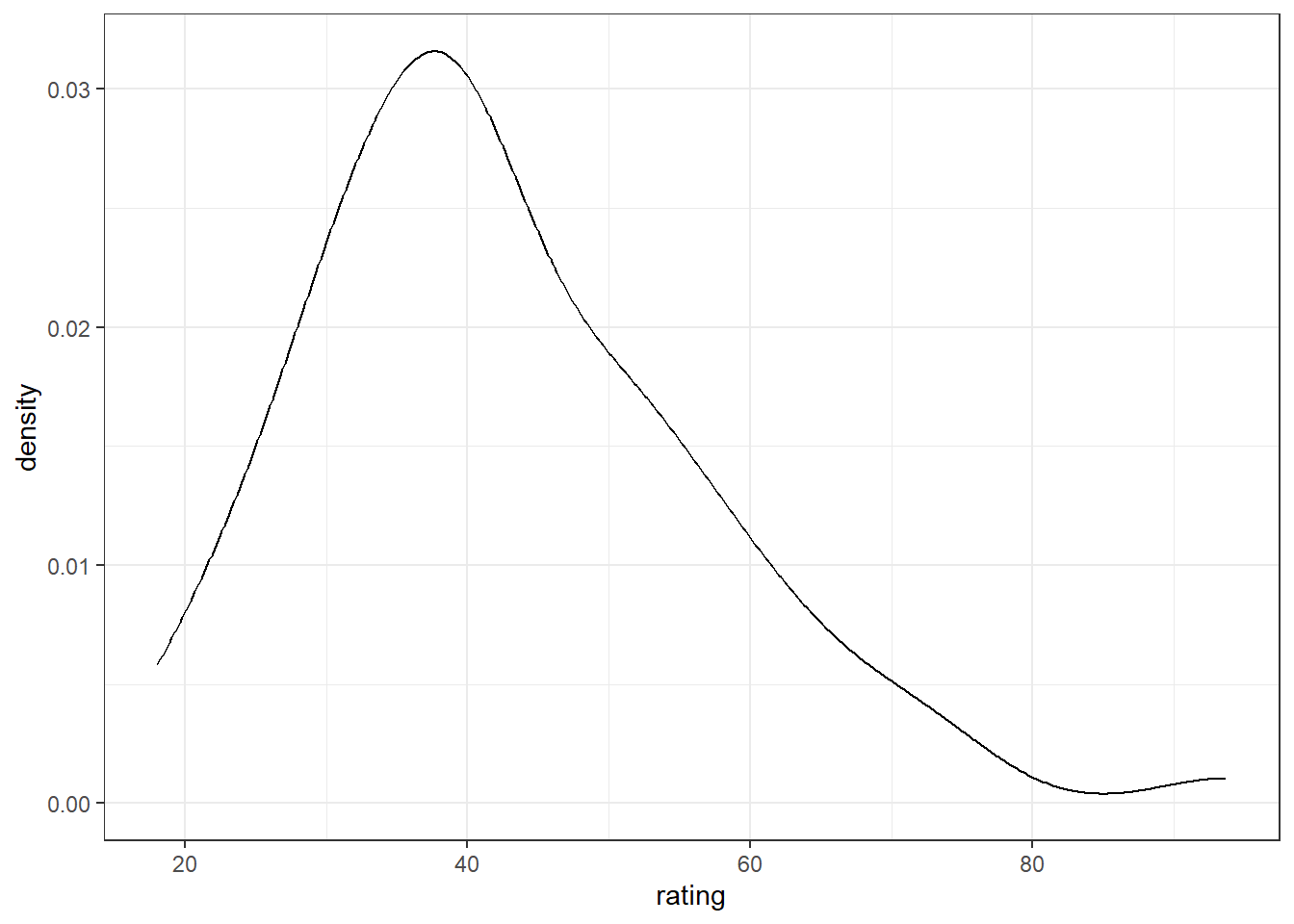Alternatively, you can put the aes(x = rating) within the geom_density() call.

ggplot(cereal) +
geom_density(aes(x = rating))#### 1b

ggplot(cereal, aes(x = rating)) +
geom_histogram()
## stat_bin() using bins = 30. Pick better value with binwidth.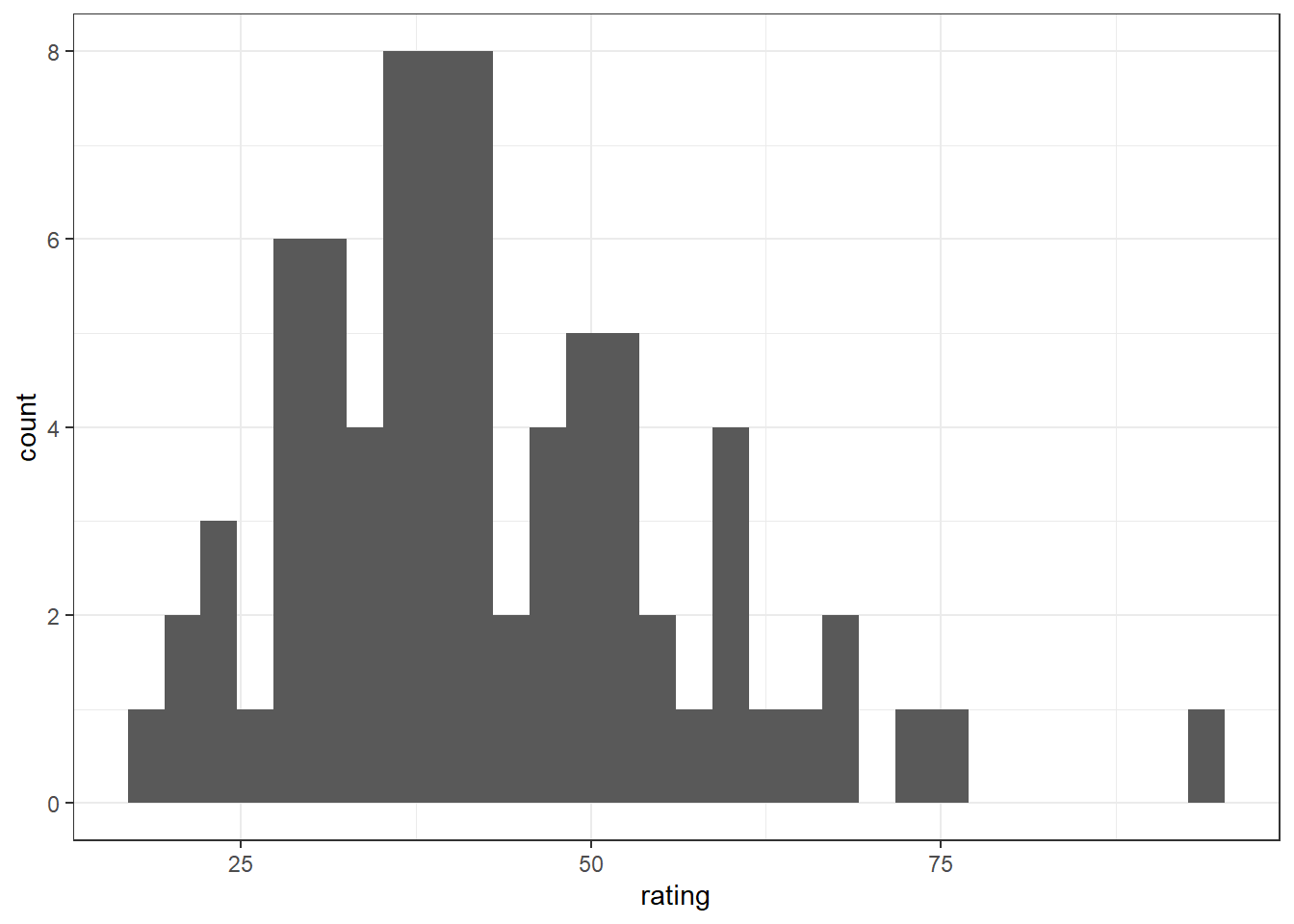#### 1c

ggplot(cereal, aes(x = rating)) +
geom_histogram(fill = 'skyblue') +
scale_y_continuous(expand = expansion(add = c(0, 1)))
## stat_bin() using bins = 30. Pick better value with binwidth.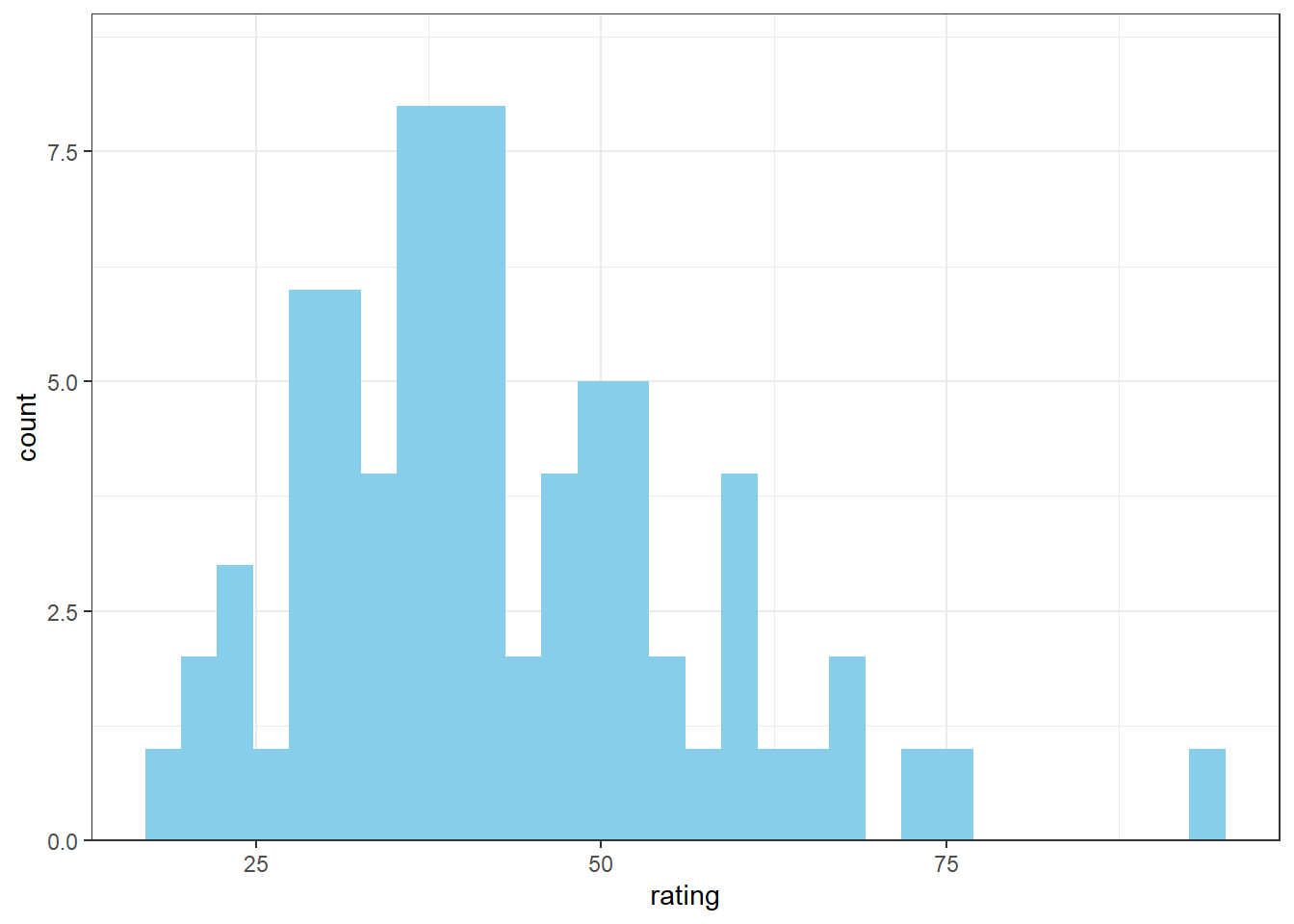Note that fill = '...' is not inside aes() because it does not map back to a variable in the data. It is just a stylistic choice. You may also choose other expansion parameters. I chose add = c(0, 1) to add 0 units of padding at the lower end and 1 unit of padding at the upper end. Unfortunately this causes non-integer breaks to appear in the y-axis by default but this could be fixed by adding, for example breaks = c(0, 4, 8) to the call to scale_y_continuous().

### Exercise 2

#### 2a

ggplot(cereal, aes(x = manufacturer, y = rating)) +
geom_boxplot()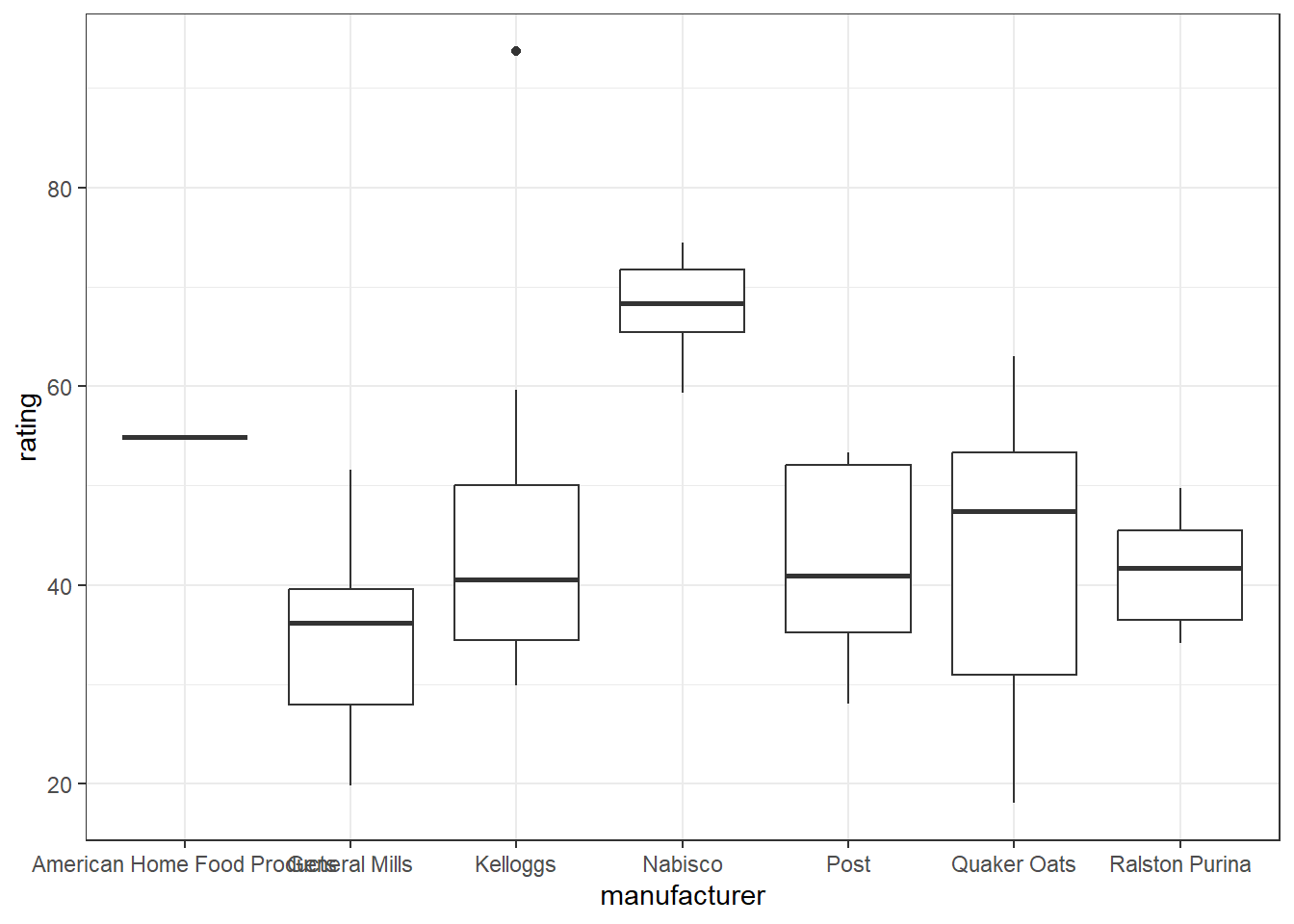#### 2b

ggplot(cereal, aes(x = manufacturer, y = rating)) +
geom_boxplot(fill = 'oldlace', outlier.shape = 1)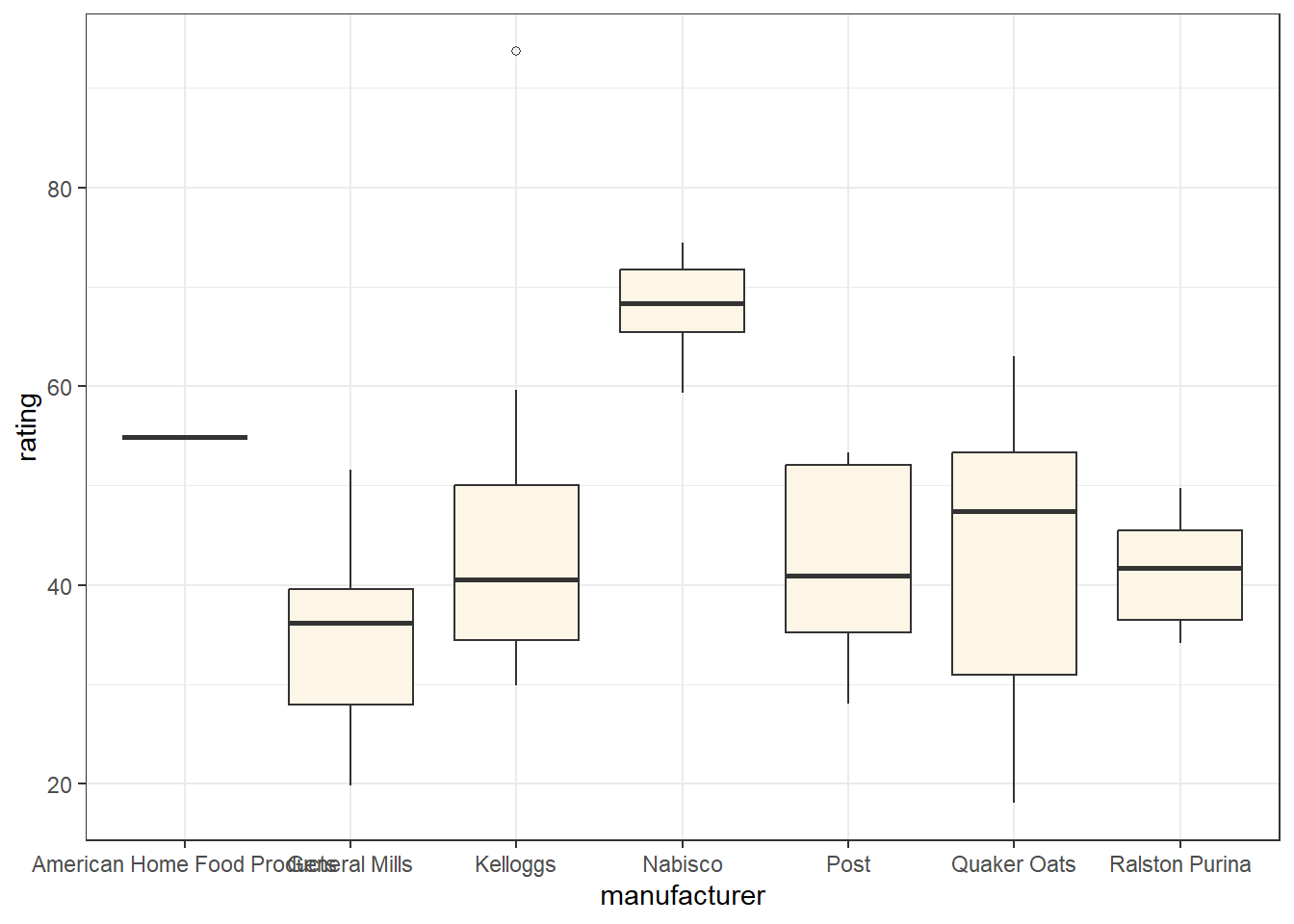Bonus content: You might’ve noticed that the names of the manufacturers are long and overlap one another. This can be fixed by customizing the x-axis labels so that the labels wrap onto multiple lines of text as follows. I used width = 15 to split lines every 15 characters, but other numbers could be used.

ggplot(cereal, aes(x = manufacturer, y = rating)) +
geom_boxplot(fill = 'oldlace', outlier.shape = 1) +
scale_x_discrete(labels = function(x) stringr::str_wrap(x, width = 15))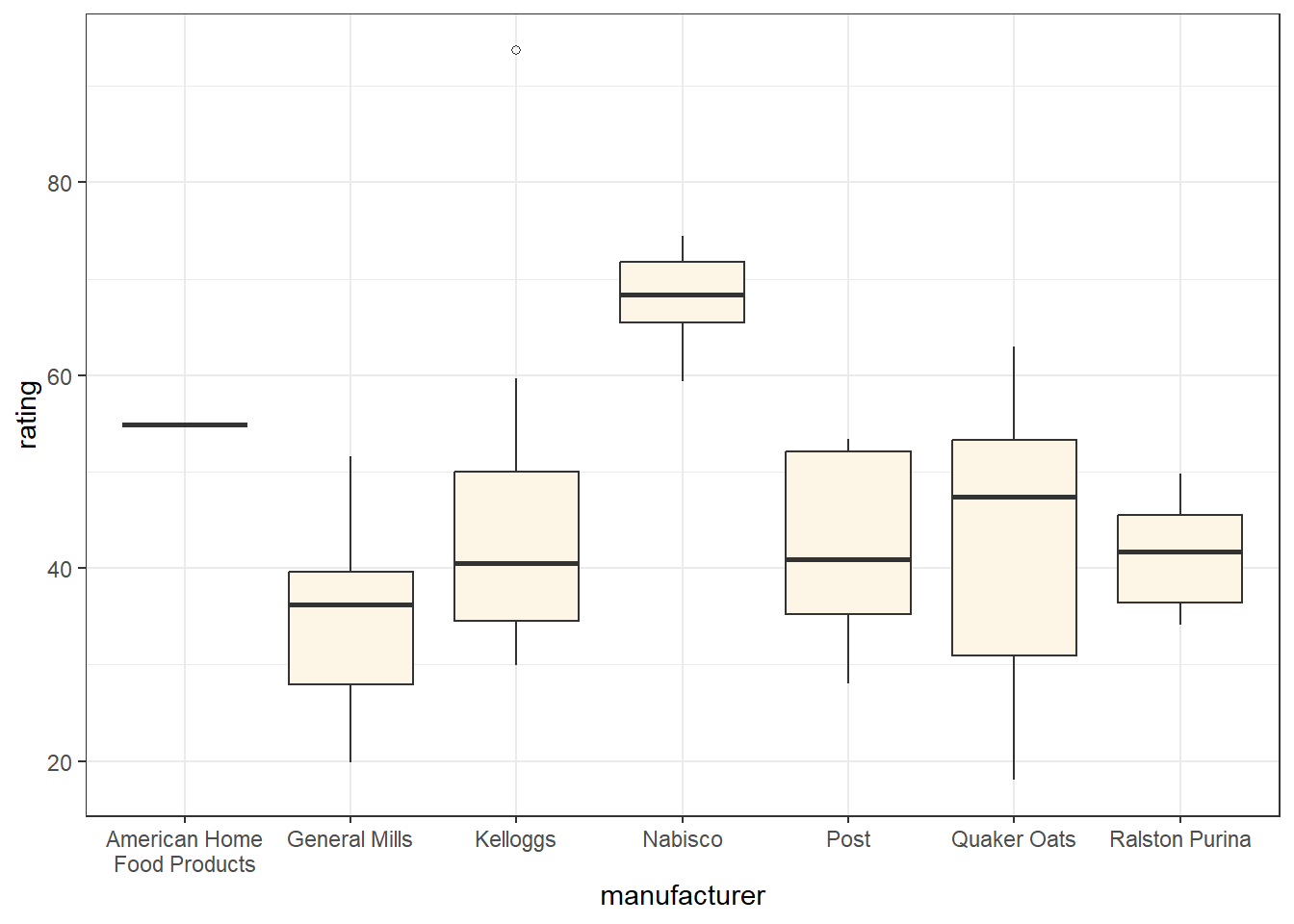### Exercise 3

#### 3a

ggplot(cereal, aes(x = sugars, y = rating)) +
geom_point()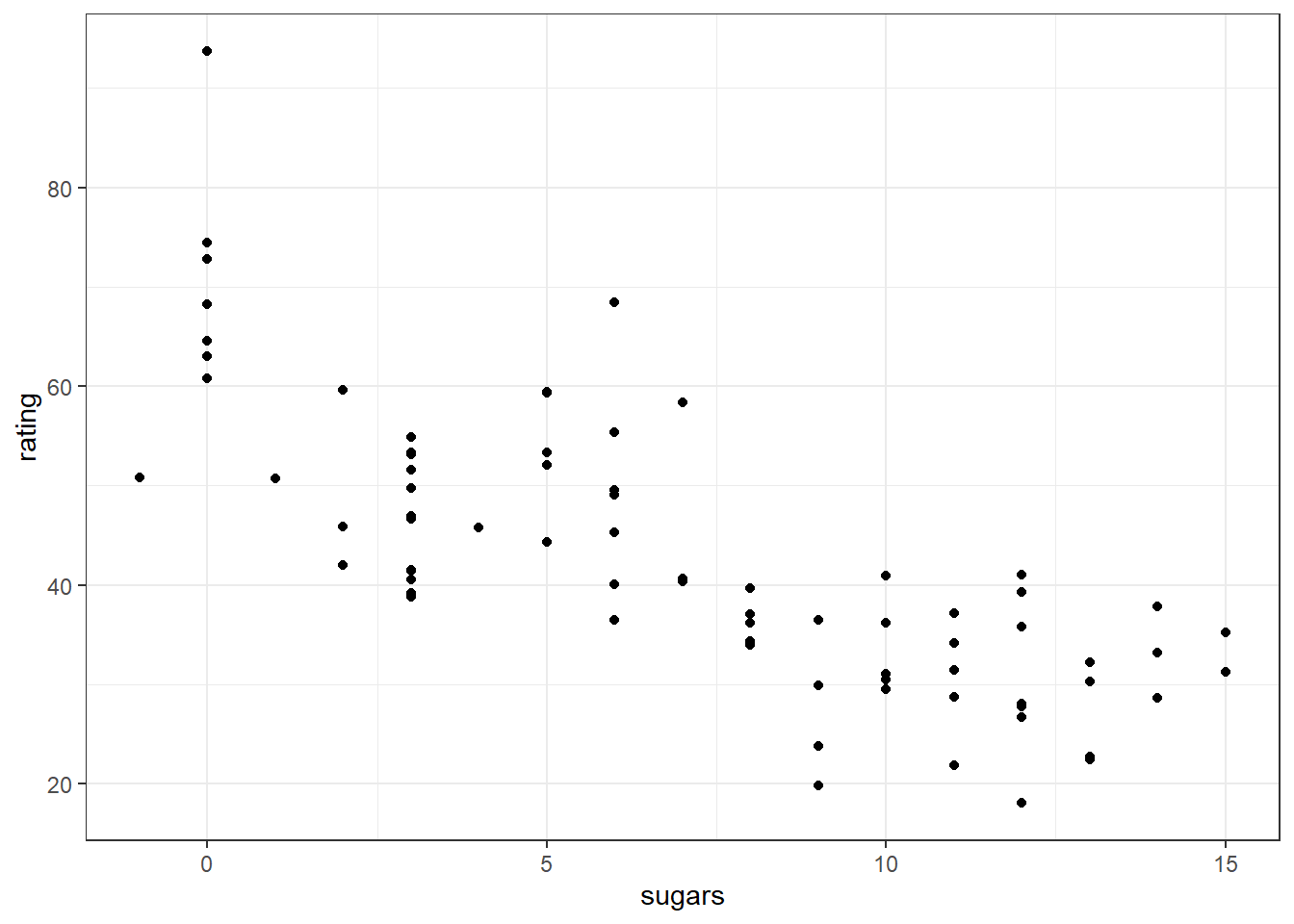#### 3b

ggplot(cereal, aes(x = sugars, y = rating)) +
geom_point() +
geom_smooth(method = lm)
## geom_smooth() using formula = 'y ~ x'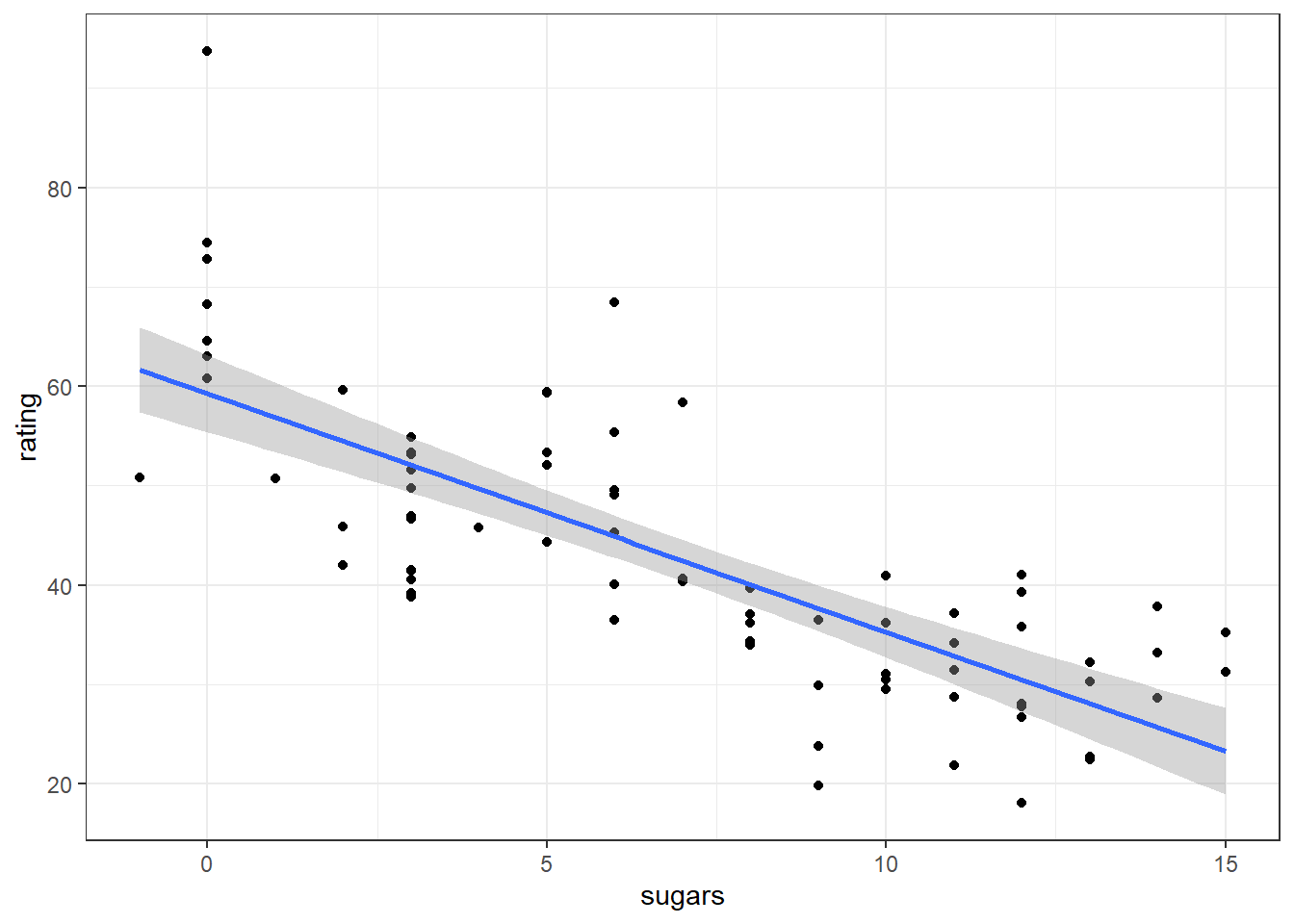The default for geom_smooth() is se = TRUE. The standard error around the trend is shown as a shaded region.

#### 3c

ggplot(cereal, aes(x = sugars, y = rating)) +
geom_point() +
geom_smooth(method = lm) +
facet_wrap( ~ type)
## geom_smooth() using formula = 'y ~ x'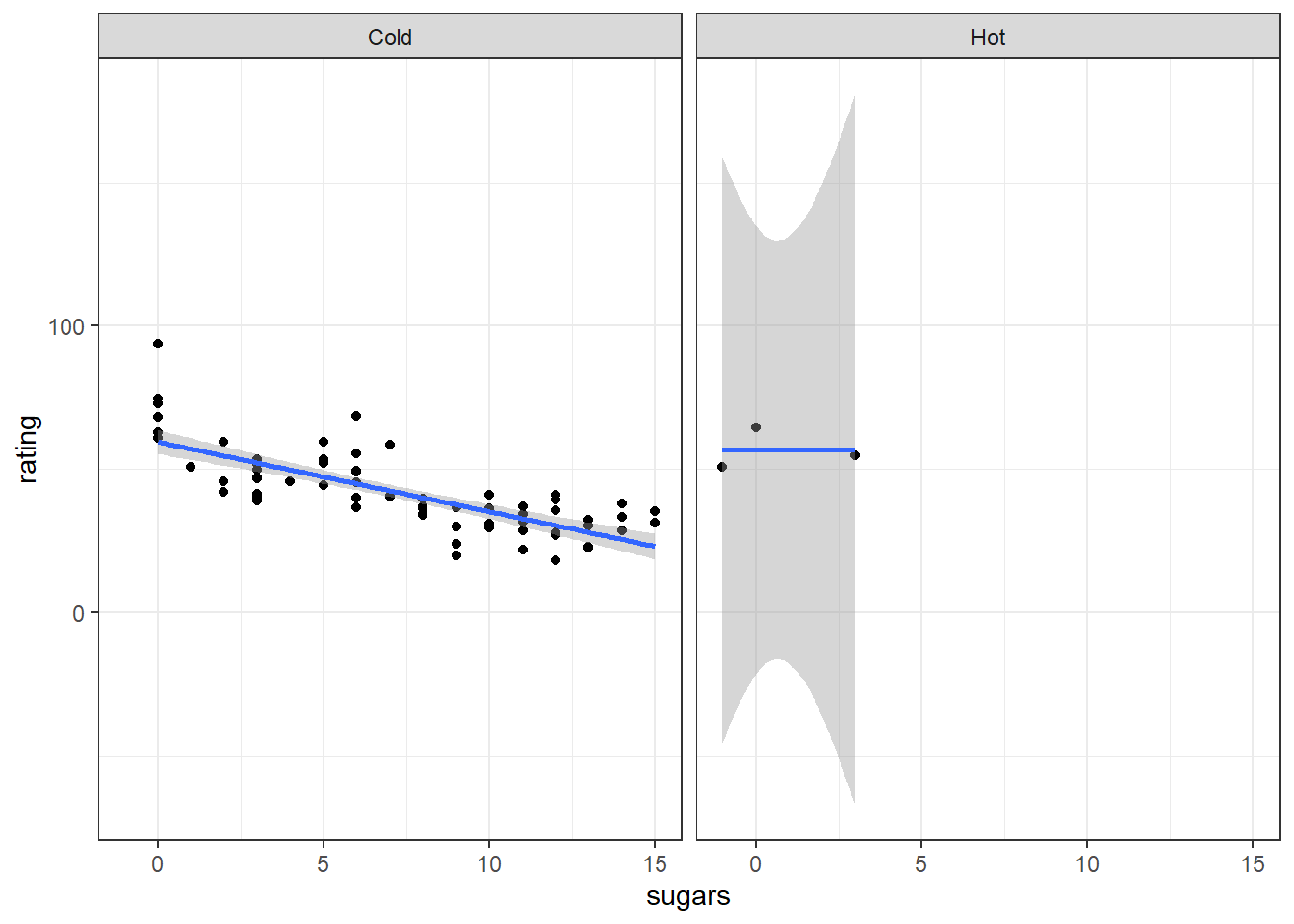Bonus content: As there are only a handful of hot cereals, all with very low sugar content and moderate to high health rating, we have a lopsided looking plot. Also, the standard errors of rating extend beyond the 0-100 range. We would have to use a special model to fix that problem if we were really doing a formal analysis here. I will fix it for plotting purposes by allowing the x-axes to vary by facet (scales = 'free_x') and using coord_cartesian() to truncate the y-axis so that the standard error envelope outside of 0-100 isn’t shown.

ggplot(cereal, aes(x = sugars, y = rating)) +
geom_point() +
geom_smooth(method = lm) +
facet_wrap(~ type, scales = 'free_x') +
coord_cartesian(ylim = c(0, 100))
## geom_smooth() using formula = 'y ~ x'